How to Select First 5 Characters from another Cell in Microsoft Excel

To select the first 5 characters from another cell in Microsoft Excel 2016/2013/2010, we need to use the “Left” function.

LEFT: Returns the specified number of characters starting from the left-most character in the text string.

Syntax of “LEFT” function:

 =LEFT (text,[num_chars])

Example: In this example Cell A2 contains a code whose first 5 characters are city code, to separate the city code from the text, we should know excel formula to copy the first 5 characters in excel: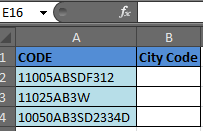=LEFT(A2, 5)

Thus, Excel matches the first 5 characters and returns “11005”, as shown in the image. Copy the formula in the cells below to do the same for other codes.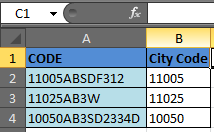This formula is the command that locates and selects specific text.

Let’s take an example to understand how to select the first 5 characters from another cell.

Example 1: I have a list of email addresses in column A. The username is the first 5 characters of the email addresses.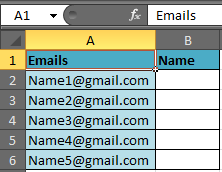To separate the first 5 characters from the list, follow the below-mentioned steps:-

Select Cell B2, write the formula

 =LEFT (A2, 5)

and press Enter on the keyboard.

The function will return the first 5 characters from the cell.

To copy the formula in all cells select cell B2, press the keys“CTRL + C” on the keyboard and select the range B3 to B6 and press key “CTRL + V”.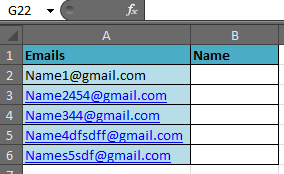Note: If there is no specific number of characters for the name in the email id, you can use the “Left” function along with the “Search” function.

SEARCH: The SEARCH function return the starting position of a text string which it locates within the specified text string.

Syntax of “SEARCH” function:

 =SEARCH (find_text,within_text,[start_num])

Use FIND instead of SEARCH if you want it to be case sensitive.

Example:

Cell A1 contains the text “Bush, George”

=SEARCH (“,”, A1, 1), the function will return 5.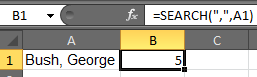This means that the “comma” is located at the 5 positions in the text string, starting from the left-most character.

To separate the name from the list by using the “Left” function along with “Search” function follow the below-mentioned steps:-

Select the Cell B2, write the formula. Press Enter on the keyboard.

 =LEFT (A2, SEARCH ("@", A2)-1).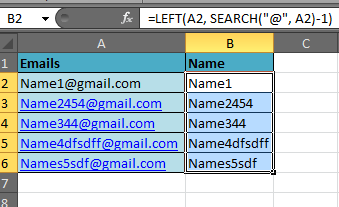The function will return the name from the email id.

Copy the formula in all cells press key “CTRL + C” on the keyboard and select the range B3 to B6 and press key “CTRL + V”.

Here we can see that the name is returned from the email id irrespective of how many characters are present in the name.

Extract Text After a Specific Character/s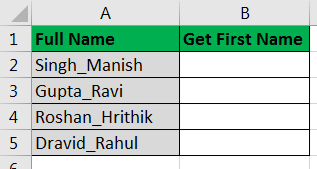Sometimes you gotta retrieve text from the right. As in the above image, you can see we have column name Full Name. It has a name in reverse order (surname_firstname). Next to name column we have a column named Get First Name. We need to extract first name from full name in Column B. Lets see the formula first:

Copy this formula in B2 and drag it down.

 =RIGHT(A2,LEN(A2)-FIND("_",A2))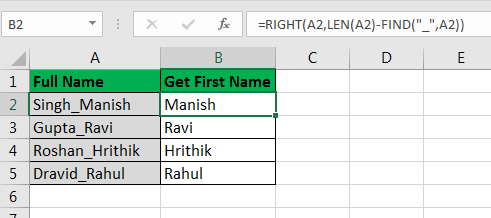Looks complicated? Its not. Let me explain.  As in all formulas, middle function is translated first (except IF), we will start from middle too.

LEN(A2): The LEN function in excel counts how many characters in a cell. It will translate to 12. Which makes our function

 =RIGHT(A2,12-FIND("_",A2))

FIND("_",A2): Similar to SEARCH function, FIND function returns the position of character/s in cell. This will translate to 6. Which makes our function

 =RIGHT(A2,12-6)  =>RIGHT(A2,6)

RIGHT(A2,6):  The RIGHT function in excel returns a specified number of characters from a cell. Here it will translate to Manish.

I tried to explain use of LEFT and RIGHT functions and how you can combine them with LEN, SEARCH and FIND function to make it dynamic. I hope it was helpful, but if it wasn’t let me know it in  the comments section.

Related Articles:

Extract Text From A String In Excel Using Excel’s LEFT And RIGHT Function

How To Extract Domain Name from EMail in Excel

Split Numbers and Text from String in Excel

Popular Articles:

50 Excel Shortcuts to Increase Your Productivity

How to use the VLOOKUP Function in Excel

How to use the COUNTIF function in Excel

How to use the SUMIF Function in Excel

1.Hello,
Could you pls help me with my problem. I have a list of customers one column in Excel.
Firstly I must find any duplicate; secondly, I should combine duplicates. The problem is there a lot of mess, for example, The Bread and Thebread, or Zinck.ru and zinckru, or Popcorns and Popcorn's. I would greatly appreciate any help.

•Hi Sophie,

Use the Search function in adjacent column to search for confirmed strings in messed up characters. Use the partial match method below.
https://www.exceltip.com/lookup-formulas/how-to-get-position-of-first-partial-match.html

2.Hello,
Can someone help me with a formula to compare characters in column B to characters in column C??
I need to compare B13 to C13, then I need to compare B14 to C14, then I need to compare B15 to C15, etc... all the way to row 3993.

Thank you,
Jessica Porras

•simply write =B13=C13 in D13 and copy this in below cells. All the relative cells will be compared automatically and TRUE-FALSE values will be shown in D13, D14,...

https://www.exceltip.com/excel-generals/relative-and-absolute-reference-in-excel.html

3.If you are trying to extract a number from a text string, I have used =abs((left (A2,5))

4.I like the helpful information you provide in your articles. Ill bookmark your weblog and check again here regularly. I'm quite certain I will learn plenty of new stuff right here! Good luck for the next!

5.Fabio Rodrigues

This formula is correct? I tried several times and it is not working.

•Hi Fabio,

Could you let us know that what are you trying to extract and what exactly are you getting?

•Yes, its correct. IT worked for me.

I did as below:

=get(E1,5)

i/p-->7035614568
o/p--> 70356

Terms and Conditions of use

The applications/code on this site are distributed as is and without warranties or liability. In no event shall the owner of the copyrights, or the authors of the applications/code be liable for any loss of profit, any problems or any damage resulting from the use or evaluation of the applications/code.